# Annex F Practical guidance on evaluating uncertainty components

This annex gives additional suggestions for evaluating uncertainty components, mainly of a practical nature, that are intended to complement the suggestions already given in Clause 4.

# F.1   Components evaluated from repeated observations: Type A evaluation of standard uncertainty

## F.1.1   Randomness and repeated observations

F.1.1.1   Uncertainties determined from repeated observations are often contrasted with those evaluated by other means as being “objective”, “statistically rigorous”, etc. That incorrectly implies that they can be evaluated merely by the application of statistical formulae to the observations and that their evaluation does not require the application of some judgement.

F.1.1.2   It must first be asked, “To what extent are the repeated observations completely independent repetitions of the measurement procedure?” If all of the observations are on a single sample, and if sampling is part of the measurement procedure because the measurand is the property of a material (as opposed to the property of a given specimen of the material), then the observations have not been independently repeated; an evaluation of a component of variance arising from possible differences among samples must be added to the observed variance of the repeated observations made on the single sample.

If zeroing an instrument is part of the measurement procedure, the instrument ought to be rezeroed as part of every repetition, even if there is negligible drift during the period in which observations are made, for there is potentially a statistically determinable uncertainty attributable to zeroing.

Similarly, if a barometer has to be read, it should in principle be read for each repetition of the measurement (preferably after disturbing it and allowing it to return to equilibrium), for there may be a variation both in indication and in reading, even if the barometric pressure is constant.

F.1.1.3   Second, it must be asked whether all of the influences that are assumed to be random really are random. Are the means and variances of their distributions constant, or is there perhaps a drift in the value of an unmeasured influence quantity during the period of repeated observations? If there is a sufficient number of observations, the arithmetic means of the results of the first and second halves of the period and their experimental standard deviations may be calculated and the two means compared with each other in order to judge whether the difference between them is statistically significant and thus if there is an effect varying with time.

F.1.1.4   If the values of “common services” in the laboratory (electric‑supply voltage and frequency, water pressure and temperature, nitrogen pressure, etc.) are influence quantities, there is normally a strongly nonrandom element in their variations that cannot be overlooked.

F.1.1.5   If the least significant figure of a digital indication varies continually during an observation due to “noise”, it is sometimes difficult not to select unknowingly personally preferred values of that digit. It is better to arrange some means of freezing the indication at an arbitrary instant and recording the frozen result.

## F.1.2   Correlations

Much of the discussion in this subclause is also applicable to Type B evaluations of standard uncertainty.

F.1.2.1   The covariance associated with the estimates of two input quantities Xi and Xj may be taken to be zero or treated as insignificant if

1. Xi and Xj are uncorrelated (the random variables, not the physical quantities that are assumed to be invariants — see 4.1.1, Note 1), for example, because they have been repeatedly but not simultaneously measured in different independent experiments or because they represent resultant quantities of different evaluations that have been made independently, or if
2. either of the quantities Xi or Xj can be treated as a constant, or if
3. there is insufficient information to evaluate the covariance associated with the estimates of Xi and Xj.

NOTE 1   On the other hand, in certain cases, such as the reference‑resistance example of Note 1 to 5.2.2, it is apparent that the input quantities are fully correlated and that the standard uncertainties of their estimates combine linearly.

NOTE 2   Different experiments may not be independent if, for example, the same instrument is used in each (see F.1.2.3).

F.1.2.2   Whether or not two repeatedly and simultaneously observed input quantities are correlated may be determined by means of Equation (17) in 5.2.3. For example, if the frequency of an oscillator uncompensated or poorly compensated for temperature is an input quantity, if ambient temperature is also an input quantity, and if they are observed simultaneously, there may be a significant correlation revealed by the calculated covariance of the frequency of the oscillator and the ambient temperature.

F.1.2.3   In practice, input quantities are often correlated because the same physical measurement standard, measuring instrument, reference datum, or even measurement method having a significant uncertainty is used in the estimation of their values. Without loss of generality, suppose two input quantities X1 and X2 estimated by x1 and x2 depend on a set of uncorrelated variables Q1Q2, ..., QL. Thus X1 = F(Q1Q2, ..., QL) and X2 = G(Q1Q2, ..., QL), although some of these variables may actually appear only in one function and not in the other. If u2(ql) is the estimated variance associated with the estimate ql of Ql, then the estimated variance associated with x1 is, from Equation (10) in 5.1.2,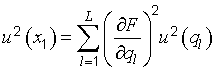(F.1)
with a similar expression for u2(x2). The estimated covariance associated with x1 and x2 is given by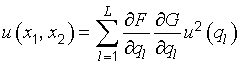(F.2)

Because only those terms for which Fql ≠ 0 and Gql ≠ 0 for a given l contribute to the sum, the covariance is zero if no variable is common to both F and G.

The estimated correlation coefficient r(x1x2) associated with the two estimates x1 and x2 is determined from u(x1x2) [Equation (F.2)] and Equation (14) in 5.2.2, with u(x1) calculated from Equation (F.1) and u(x2) from a similar expression. [See also Equation (H.9) in H.2.3.] It is also possible for the estimated covariance associated with two input estimates to have both a statistical component [see Equation (17) in 5.2.3] and a component arising as discussed in this subclause.

EXAMPLE 1   A standard resistor RS is used in the same measurement to determine both a current I and a temperature t. The current is determined by measuring, with a digital voltmeter, the potential difference across the terminals of the standard; the temperature is determined by measuring, with a resistance bridge and the standard, the resistance Rt(t) of a calibrated resistive temperature sensor whose temperature-resistance relation in the range 15 °C ≤ t ≤ 30 °C is t = aR2t(t) − t0, where a and t0 are known constants. Thus the current is determined through the relation I = VSRS and the temperature through the relation t = 2(t)R2S − t0, where β(t) is the measured ratio Rt(t)RS provided by the bridge.

Since only the quantity RS is common to the expression for I and t, Equation (F.2) yields for the covariance of I and t(For simplicity of notation, in this example the same symbol is used for both the input quantity and its estimate.)

To obtain the numerical value of the covariance, one substitutes into this expression the numerical values of the measured quantities I and t, and the values of RS and u(RS) given in the standard resistor's calibration certificate. The unit of u(It) is clearly A·°C since the dimension of the relative variance [u(RS)RS]2 is one (that is, the latter is a so-called dimensionless quantity).

Further, let a quantity P be related to the input quantities I and t by P = C0I2(T0 + t), where C0 and T0 are known constants with negligible uncertainties [u2(C0) ≈ 0. u2(T0) ≈ 0]. Equation (13) in 5.2.2 then yields for the variance of P in terms of the variances of I and t and their covariance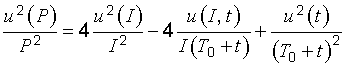The variances u2(I) and u2(t) are obtained by the application of Equation (10) of 5.1.2 to the relations I = VSRS and t = aβ2(t)R2S − t0. The results arewhere for simplicity it is assumed that the uncertainties of the constants t0 and a are also negligible. These expressions can be readily evaluated since u2(VS) and u2(β) may be determined, respectively, from the repeated readings of the voltmeter and of the resistance bridge. Of course, any uncertainties inherent in the instruments themselves and in the measurement procedures employed must also be taken into account when u2(VS) and u2(β) are determined.

EXAMPLE 2   In the example of Note 1 to 5.2.2, let the calibration of each resistor be represented by Ri = αiRS, with u(αi) the standard uncertainty of the measured ratio αi as obtained from repeated observations. Further, let αi ≈ 1 for each resistor, and let u(αi) be essentially the same for each calibration so that u(αi) ≈ u(α). Then Equations (F.1) and (F.2) yield u2(Ri) = R2Su2(α) + u2(RS) and u(RiRj) = u2(RS). This implies through Equation (14) in 5.2.2 that the correlation coefficient of any two resistors (i ≠ j) isSince u(RS)RS = 10−4, if u(α) = 100 × 10−6, rij ≈ 0,5; if u(α) = 10 × 10−6, rij ≈ 0,990; and if u(α) = 1 × 10−6, rij ≈ 1,000. Thus as u(α) → 0, rij → 1, and u(Ri) → u(RS).

NOTE   In general, in comparison calibrations such as this example, the estimated values of the calibrated items are correlated, with the degree of correlation depending upon the ratio of the uncertainty of the comparison to the uncertainty of the reference standard. When, as often occurs in practice, the uncertainty of the comparison is negligible with respect to the uncertainty of the standard, the correlation coefficients are equal to +1 and the uncertainty of each calibrated item is the same as that of the standard.

F.1.2.4   The need to introduce the covariance u(xixj) can be bypassed if the original set of input quantities X1X2, ..., XN upon which the measurand Y depends [see Equation (1) in 4.1] is redefined in such a way as to include as additional independent input quantities those quantities Ql that are common to two or more of the original Xi. (It may be necessary to perform additional measurements to establish fully the relationship between Ql and the affected Xi.) Nonetheless, in some situations it may be more convenient to retain covariances rather than to increase the number of input quantities. A similar process can be carried out on the observed covariances of simultaneous repeated observations [see Equation (17) in 5.2.3], but the identification of the appropriate additional input quantities is often ad hoc and nonphysical.

EXAMPLE   If, in Example 1 of F.1.2.3, the expressions for I and t in terms of RS are introduced into the expression for P, the result isand the correlation between I and t is avoided at the expense of replacing the input quantities I and t with the quantities VS, RS, and β. Since these quantities are uncorrelated, the variance of P can be obtained from Equation (10) in 5.1.2.

# F.2   Components evaluated by other means: Type B evaluation of standard uncertainty

## F.2.1   The need for Type B evaluations

If a measurement laboratory had limitless time and resources, it could conduct an exhaustive statistical investigation of every conceivable cause of uncertainty, for example, by using many different makes and kinds of instruments, different methods of measurement, different applications of the method, and different approximations in its theoretical models of the measurement. The uncertainties associated with all of these causes could then be evaluated by the statistical analysis of series of observations and the uncertainty of each cause would be characterized by a statistically evaluated standard deviation. In other words, all of the uncertainty components would be obtained from Type A evaluations. Since such an investigation is not an economic practicality, many uncertainty components must be evaluated by whatever other means is practical.

## F.2.2   Mathematically determinate distributions

### F.2.2.1   The resolution of a digital indication

One source of uncertainty of a digital instrument is the resolution of its indicating device. For example, even if the repeated indications were all identical, the uncertainty of the measurement attributable to repeatability would not be zero, for there is a range of input signals to the instrument spanning a known interval that would give the same indication. If the resolution of the indicating device is δx, the value of the stimulus that produces a given indication X can lie with equal probability anywhere in the interval X − δx2 to X + δx2. The stimulus is thus described by a rectangular probability distribution (see 4.3.7 and 4.4.5) of width δx with variance u2 = (δx)212, implying a standard uncertainty of u = 0,29δx for any indication.

Thus a weighing instrument with an indicating device whose smallest significant digit is 1 g has a variance due to the resolution of the device of u2 = (112) g2 and a standard uncertainty of u = (112‾‾‾‾‾) g = 0,29 g.

### F.2.2.2   Hysteresis

Certain kinds of hysteresis can cause a similar kind of uncertainty. The indication of an instrument may differ by a fixed and known amount according to whether successive readings are rising or falling. The prudent operator takes note of the direction of successive readings and makes the appropriate correction. But the direction of the hysteresis is not always observable: there may be hidden oscillations within the instrument about an equilibrium point so that the indication depends on the direction from which that point is finally approached. If the range of possible readings from that cause is δx, the variance is again u2 = (δx)212 and the standard uncertainty due to hysteresis is u = 0,29δx.

### F.2.2.3   Finite‑precision arithmetic

The rounding or truncation of numbers arising in automated data reduction by computer can also be a source of uncertainty. Consider, for example, a computer with a word length of 16 bits. If, in the course of computation, a number having this word length is subtracted from another from which it differs only in the 16th bit, only one significant bit remains. Such events can occur in the evaluation of “ill-conditioned” algorithms, and they can be difficult to predict. One may obtain an empirical determination of the uncertainty by increasing the most important input quantity to the calculation (there is frequently one that is proportional to the magnitude of the output quantity) by small increments until the output quantity changes; the smallest change in the output quantity that can be obtained by such means may be taken as a measure of the uncertainty; if it is δx, the variance is u2 = (δx)212 and u = 0,29δx.

NOTE   One may check the uncertainty evaluation by comparing the result of the computation carried out on the limited word‑length machine with the result of the same computation carried out on a machine with a significantly larger word length.

## F.2.3   Imported input values

F.2.3.1   An imported value for an input quantity is one that has not been estimated in the course of a given measurement but has been obtained elsewhere as the result of an independent evaluation. Frequently such an imported value is accompanied by some kind of statement about its uncertainty. For example, the uncertainty may be given as a standard deviation, a multiple of a standard deviation, or the half-width of an interval having a stated level of confidence. Alternatively, upper and lower bounds may be given, or no information may be provided about the uncertainty. In the latter case, those who use the value must employ their own knowledge about the likely magnitude of the uncertainty, given the nature of the quantity, the reliability of the source, the uncertainties obtained in practice for such quantities, etc.

NOTE   The discussion of the uncertainty of imported input quantities is included in this subclause on Type B evaluation of standard uncertainty for convenience; the uncertainty of such a quantity could be composed of components obtained from Type A evaluations or components obtained from both Type A and Type B evaluations. Since it is unnecessary to distinguish between components evaluated by the two different methods in order to calculate a combined standard uncertainty, it is unnecessary to know the composition of the uncertainty of an imported quantity.

F.2.3.2   Some calibration laboratories have adopted the practice of expressing “uncertainty” in the form of upper and lower limits that define an interval having a “minimum” level of confidence, for example, “at least” 95 percent. This may be viewed as an example of a so-called “safe” uncertainty (see E.1.2), and it cannot be converted to a standard uncertainty without a knowledge of how it was calculated. If sufficient information is given, it may be recalculated in accordance with the rules of this Guide; otherwise an independent assessment of the uncertainty must be made by whatever means are available.

F.2.3.3   Some uncertainties are given simply as maximum bounds within which all values of the quantity are said to lie. It is a common practice to assume that all values within those bounds are equally probable (a rectangular probability distribution), but such a distribution should not be assumed if there is reason to expect that values within but close to the bounds are less likely than those nearer the centre of the bounds. A rectangular distribution of half‑width a has a variance of a23; a normal distribution for which a is the half‑width of an interval having a level of confidence of 99,73 percent has a variance of a29. It may be prudent to adopt a compromise between those values, for example, by assuming a triangular distribution for which the variance is a26 (see 4.3.9 and 4.4.6).

## F.2.4   Measured input values

### F.2.4.1   Single observation, calibrated instruments

If an input estimate has been obtained from a single observation with a particular instrument that has been calibrated against a standard of small uncertainty, the uncertainty of the estimate is mainly one of repeatability. The variance of repeated measurements by the instrument may have been obtained on an earlier occasion, not necessarily at precisely the same value of the reading but near enough to be useful, and it may be possible to assume the variance to be applicable to the input value in question. If no such information is available, an estimate must be made based on the nature of the measuring apparatus or instrument, the known variances of other instruments of similar construction, etc.

### F.2.4.2   Single observation, verified instruments

Not all measuring instruments are accompanied by a calibration certificate or a calibration curve. Most instruments, however, are constructed to a written standard and verified, either by the manufacturer or by an independent authority, to conform to that standard. Usually the standard contains metrological requirements, often in the form of “maximum permissible errors”, to which the instrument is required to conform. The compliance of the instrument with these requirements is determined by comparison with a reference instrument whose maximum allowed uncertainty is usually specified in the standard. This uncertainty is then a component of the uncertainty of the verified instrument.

If nothing is known about the characteristic error curve of the verified instrument it must be assumed that there is an equal probability that the error has any value within the permitted limits, that is, a rectangular probability distribution. However, certain types of instruments have characteristic curves such that the errors are, for example, likely always to be positive in part of the measuring range and negative in other parts. Sometimes such information can be deduced from a study of the written standard.

### F.2.4.3   Controlled quantities

Measurements are frequently made under controlled reference conditions that are assumed to remain constant during the course of a series of measurements. For example, measurements may be performed on specimens in a stirred oil bath whose temperature is controlled by a thermostat. The temperature of the bath may be measured at the time of each measurement on a specimen, but if the temperature of the bath is cycling, the instantaneous temperature of the specimen may not be the temperature indicated by the thermometer in the bath. The calculation of the temperature fluctuations of the specimen based on heat‑transfer theory, and of their variance, is beyond the scope of this Guide, but it must start from a known or assumed temperature cycle for the bath. That cycle may be observed by a fine thermocouple and a temperature recorder, but failing that, an approximation of it may be deduced from a knowledge of the nature of the controls.

### F.2.4.4   Asymmetric distributions of possible values

There are occasions when all possible values of a quantity lie to one side of a single limiting value. For example, when measuring the fixed vertical height h (the measurand) of a column of liquid in a manometer, the axis of the height‑measuring device may deviate from verticality by a small angle β. The distance l determined by the device will always be larger than h; no values less than h are possible. This is because h is equal to the projection lcosβ, implying l = hcosβ, and all values of cosβ are less than one; no values greater than one are possible. This so‑called “cosine error” can also occur in such a way that the projection hcosβ of a measurand h is equal to the observed distance l, that is, l = hcosβ, and the observed distance is always less than the measurand.

If a new variable δ = 1 − cosβ is introduced, the two different situations are, assuming β ≈ 0 or δ ≪ 1 as is usually the case in practice,(F.3a)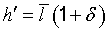(F.3b)

Here l‾‾, the best estimate of l, is the arithmetic mean or average of n independent repeated observations lk of l with estimated variance u2(l‾‾) [see Equations (3) and (5) in 4.2]. Thus it follows from Equations (F.3a) and (F.3b) that to obtain an estimate of h or h requires an estimate of the correction factor δ, while to obtain the combined standard uncertainty of the estimate of h or h requires u2(δ), the estimated variance of δ. More specifically, application of Equation (10) in 5.1.2 to Equations (F.3a) and (F.3b) yields for u2c(h) and u2c(h) (− and + signs, respectively)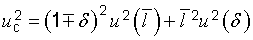(F.4a)(F.4b)

To obtain estimates of the expected value of δ and the variance of δ, assume that the axis of the device used to measure the height of the column of liquid in the manometer is constrained to be fixed in a vertical plane and that the distribution of the values of the angle of inclination β about its expected value of zero is a normal distribution with variance σ2. Although β can have both positive and negative values, δ = 1 − cosβ is positive for all values of β. If the misalignment of the axis of the device is assumed to be unconstrained, the orientation of the axis can vary over a solid angle since it is capable of misalignment in azimuth as well, but β is then always a positive angle.

In the constrained or one‑dimensional case, the probability element p(β)dβ (C.2.5, note) is proportional to {exp[− β2(2σ2)]}dβ; in the unconstrained or two‑dimensional case, the probability element is proportional to {exp[− β2(2σ2)]}sinβ dβ. The probability density functions p(δ) in the two cases are the expressions required to determine the expectation and variance of δ for use in Equations (F.3) and (F.4). They may readily be obtained from these probability elements because the angle β may be assumed small, and hence δ = 1 − cosβ and sinβ may be expanded to lowest order in β. This yields δ ≈ β22, sinβ ≈ β = 2δ‾‾‾‾, and dβ = dδ2δ‾‾‾‾. The probability density functions are then(F.5a)

in one dimension(F.5b)

in two dimensions

whereEquations (F.5a) and (F.5b), which show that the most probable value of the correction δ in both cases is zero, give in the one-dimensional case E(δ) = σ22 and var(δ) = σ42 for the expectation and the variance of δ; and in the two‑dimensional case E(δ) = σ2 and var(δ) = σ4. Equations (F.3a), (F.3b), and (F.4b) become then(F.6a)(F.6b)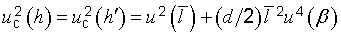(F.6c)
where d is the dimensionality (d = 1 or 2) and u(β) is the standard uncertainty of the angle β, taken to be the best estimate of the standard deviation σ of an assumed normal distribution and to be evaluated from all of the information available concerning the measurement (Type B evaluation). This is an example of a case where the estimate of the value of a measurand depends on the uncertainty of an input quantity.

Although Equations (F.6a) to (F.6c) are specific to the normal distribution, the analysis can be carried out assuming other distributions for β. For example, if one assumes for β a symmetric rectangular distribution with upper and lower bounds of +β0 and β0 in the one‑dimensional case and +β0 and zero in the two‑dimensional case, E(δ) = β206 and var(δ) = β4045 in one dimension; and E(δ) = β204 and var(δ) = β4048 in two dimensions.

NOTE   This is a situation where the expansion of the function Y = f(X1X2, ..., XN) in a first‑order Taylor series to obtain u2c(y), Equation (10) in 5.1.2, is inadequate because of the nonlinearity of f: cosβ‾‾‾‾‾‾‾‾ ≠ cosβ‾‾ (see Note to 5.1.2, and H.2.4). Although the analysis can be carried out entirely in terms of β, introducing the variable δ simplifies the problem.

Another example of a situation where all possible values of a quantity lie to one side of a single limiting value is the determination by titration of the concentration of a component in a solution where the end point is indicated by the triggering of a signal; the amount of reagent added is always more than that necessary to trigger the signal; it is never less. The excess titrated beyond the limit point is a required variable in the data reduction, and the procedure in this (and in similar) cases is to assume an appropriate probability distribution for the excess and to use it to obtain the expected value of the excess and its variance.

EXAMPLE   If a rectangular distribution of lower bound zero and upper bound C0 is assumed for the excess z, then the expected value of the excess is C02 with associated variance C2012. If the probability density function of the excess is taken as that of a normal distribution with 0 ≤ z < ∞, that is, p(z) = (σπ/2‾‾‾‾‾‾‾)−1exp[(−z22σ2)], then the expected value is σ2/π‾‾‾‾‾‾ with variance σ2(1 − 2/π).

### F.2.4.5   Uncertainty when corrections from a calibration curve are not applied

The note to 6.3.1 discusses the case where a known correction b for a significant systematic effect is not applied to the reported result of a measurement but instead is taken into account by enlarging the “uncertainty” assigned to the result. An example is replacement of an expanded uncertainty U with U + b, where U is an expanded uncertainty obtained under the assumption b = 0. This practice is sometimes followed in situations where all of the following conditions apply: the measurand Y is defined over a range of values of a parameter t, as in the case of a calibration curve for a temperature sensor; U and b also depend on t; and only a single value of “uncertainty” is to be given for all estimates y(t) of the measurand over the range of possible values of t. In such situations the result of the measurement is often reported as Y(t) = y(t) ± [Umax + bmax], where the subscript “max” indicates that the maximum value of U and the maximum value of the known correction b over the range of values of t are used.

Although this Guide recommends that corrections be applied to measurement results for known significant systematic effects, this may not always be feasible in such a situation because of the unacceptable expense that would be incurred in calculating and applying an individual correction, and in calculating and using an individual uncertainty, for each value of y(t).

A comparatively simple approach to this problem that is consistent with the principles of this Guide is as follows:

Compute a single mean correction b‾‾ from(F.7a)
where t1 and t2 define the range of interest of the parameter t, and take the best estimate of Y(t) to be y(t) = y(t) + b‾‾, where y(t) is the best uncorrected estimate of Y(t). The variance associated with the mean correction b‾‾ over the range of interest is given by(F.7b)
not taking into account the uncertainty of the actual determination of the correction b(t). The mean variance of the correction b(t) due to its actual determination is given by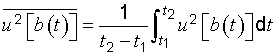(F.7c)
where u2[b(t)] is the variance of the correction b(t). Similarly, the mean variance of y(t) arising from all sources of uncertainty other than the correction b(t) is obtained from(F.7d)
where u2[y(t)] is the variance of y(t) due to all uncertainty sources other than b(t). The single value of standard uncertainty to be used for all estimates y(t) = y(t) + b‾‾ of the measurand Y(t) is then the positive square root of(F.7e)

An expanded uncertainty U may be obtained by multiplying uc(y) by an appropriately chosen coverage factor k, U = kuc(y), yielding Y(t) = y(t) ± U = y(t) + b‾‾ ± U. However, the use of the same average correction for all values of t rather than the correction appropriate for each value of t must be recognized and a clear statement given as to what U represents.

## F.2.5   Uncertainty of the method of measurement

F.2.5.1   Perhaps the most difficult uncertainty component to evaluate is that associated with the method of measurement, especially if the application of that method has been shown to give results with less variability than those of any other that is known. But it is likely that there are other methods, some of them as yet unknown or in some way impractical, that would give systematically different results of apparently equal validity. This implies an a priori probability distribution, not a distribution from which samples can be readily drawn and treated statistically. Thus, even though the uncertainty of the method may be the dominant one, the only information often available for evaluating its standard uncertainty is one's existing knowledge of the physical world. (See also E.4.4.)

NOTE   Determining the same measurand by different methods, either in the same laboratory or in different laboratories, or by the same method in different laboratories, can often provide valuable information about the uncertainty attributable to a particular method. In general, the exchange of measurement standards or reference materials between laboratories for independent measurement is a useful way of assessing the reliability of evaluations of uncertainty and of identifying previously unrecognized systematic effects.

## F.2.6   Uncertainty of the sample

F.2.6.1   Many measurements involve comparing an unknown object with a known standard having similar characteristics in order to calibrate the unknown. Examples include end gauges, certain thermometers, sets of masses, resistors, and high purity materials. In most such cases, the measurement methods are not especially sensitive to, or adversely affected by, sample selection (that is, the particular unknown being calibrated), sample treatment, or the effects of various environmental influence quantities because the unknown and standard respond in generally the same (and often predictable) way to such variables.

F.2.6.2   In some practical measurement situations, sampling and specimen treatment play a much larger role. This is often the case for the chemical analysis of natural materials. Unlike man-made materials, which may have proven homogeneity to a level beyond that required for the measurement, natural materials are often very inhomogeneous. This inhomogeneity leads to two additional uncertainty components. Evaluation of the first requires determining how adequately the sample selected represents the parent material being analysed. Evaluation of the second requires determining the extent to which the secondary (unanalysed) constituents influence the measurement and how adequately they are treated by the measurement method.

F.2.6.3   In some cases, careful design of the experiment may make it possible to evaluate statistically the uncertainty due to the sample (see H.5 and H.5.3.2). Usually, however, especially when the effects of environmental influence quantities on the sample are significant, the skill and knowledge of the analyst derived from experience and all of the currently available information are required for evaluating the uncertainty.